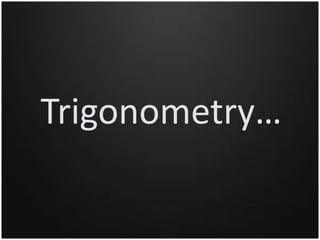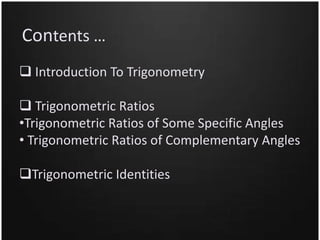Seu SlideShare está sendo baixado. ×

# Introduction To Trigonometry

Anúncio
Anúncio
Anúncio
Anúncio
Anúncio
Anúncio
Anúncio
Anúncio
Anúncio
Anúncio
AnúncioCarregando em…3
×

1 de 15 Anúncio

# Introduction To Trigonometry

It is a ppt on Trigonometry for th students of class 10 .
The basic concepts of trigonometry are provided here with examples Hope that that you like it .!! Thankyou ..!! :)

It is a ppt on Trigonometry for th students of class 10 .
The basic concepts of trigonometry are provided here with examples Hope that that you like it .!! Thankyou ..!! :)

Anúncio
Anúncio

### Introduction To Trigonometry

1. 1. New Era Progressive School Session 2015-16 PowerPoint Presentation Mathematics Submitted By : Priyanka Sahu Submitted To : Mrs. Mona Thakur
2. 2. Trigonometry…
3. 3. Contents …  Introduction To Trigonometry  Trigonometric Ratios •Trigonometric Ratios of Some Specific Angles • Trigonometric Ratios of Complementary Angles Trigonometric Identities
4. 4. Introduction To Trigonometry • Trigonometry is a branch of mathematics which deals with the measurement of the sides and the angles of a triangle and the problems allied with angles. • The word ‘Trigonometry’ is derived from the Greek words TRIGONON (means triangle) and METRON (means measure).
5. 5. Trigonometric Ratios • Let us consider a right triangle ABC : • Here angle A is an acute angle. Note the position of the side BC with respect to angle A. We will call it the perpendicular to angle A. AC is the hypotenuse of the right angled ∆ and the side AB is the part of angle A. So, we will call it as the base to angle A. • The trigonometric ratios of angle A in right angle ABC are defined as follows : • Sine of angle A = Perpendicular = BC Hypotenuse AC • Cosine of angle A = Base = AB Hypotenuse AC • Tangent of angle A = Perpendicular = BC Base AB
6. 6. • Cosecant of angle A = Hypotenuse = AC Perpendicular BC • Secant of angle A = Hypotenuse = AC Base AB • Cotangent of angle A = Base ____ =AB Perpendicular AC • The ratios defined above are abbreviated as sin A, cos A, tan A ,cosec A, sec A, and cot A respectively. Note that the ratios cosec A , sec A and cot A are respectively, the reciprocals of the ratios sin A, cos A, and tan A. • Also, observe that tan A= BC/AC = sin A and AB/AC cos A cot A = AB/AC = cos A BC/AC sin A So, the trigonometric ratios of an acute angle in a right –angled triangle express the relationship between the angle and the length of its sides.
7. 7. Example Q. In a triangle ABC, right angled at a , if AB=12cm, AC=5cm and BC=13cm ,find all the six trigonometric ratios of angle B . Sol. With reference to angle B ,we have Base=AB=12,Perpendicular=AC=5,Hypotenuse=BC=5cm Using the definitions of trigonometric ratios, we have sin B = AC/BC = 5/13 cos B = AB/BC = 12/13 tan B = AC/AB =5/12 cosec B = BC/AC = 13/5 sec B = BC/AB = 13/12 cot B = AB/AC =12/5
8. 8. Trigonometric Ratios of Some Specific Angles
9. 9. Example… Q. If x=30°,verify that cos3x=4cos³x-3cosx Sol. We have, x = 30° ⇒ 3x = 90° Cos3x = cos90°=0 And, 4cos³x - 3cosx = 4cos³30°- 3cos30° ⇒ 4cos³x – 3cosx = 4 (√3/2)³ - 3(√3/2) =4 X 3√3/8 - 3√3/2 =3√3/2 - 3√3/2 =0
10. 10. Trigonometric ratios of Complementary Angles • Complementary angle : Two angles are said to be complementary, if their sum is 90°. • It follows from the above definition that θ and (90° - θ ) are complementary angles for an acute angle θ. • So, if θ is an acute angle, then sin (90° – θ) = cos θ, cos (90° – θ) = sin θ, tan (90° – θ) = cot θ, cot (90° – θ) = tan θ, sec (90° – θ) = cosec θ, cosec (90° – θ) = sec θ,
11. 11. Example Q. Prove that : tan10°tan15°tan75°tan80° = 1 We have, LHS =tan10°tan15°tan75°tan80° = tan(90° - 80°) tan (90° - 75°) tan75°tan80° = cot80° cot75° tan75° tan80° = (cot80° tan80°) (cot75° tan75°) = 1 x 1 =1 Sol.
12. 12. Trigonometric Identities • An equation involving trigonometric ratios of an angle θ (say) is said to be a trigonometric identity if it is satisfied for all values of θ for which the given trigonometric ratios are defined. • We can obtain some more identities by using some fundamental trigonometric identities.
13. 13. 1) cos2 θ + sin2 θ = 1 2) cos2 θ =1 - sin2 θ 3) sin2 θ =1 - cos2 θ 4) 1 + tan2 θ = sec2 θ 5) sec2 θ - tan2 θ = 1 6) tan2 θ = sec2 θ – 1 7) cot2 θ + 1 = cosec2 θ 8) cosec2 θ - cot2 θ = 1 9) cot2 θ = cosec2 θ – 1 Some Fundamental Trigonometric Identities
14. 14. Example Q. Sol. Q. Sol.
15. 15. Thankyou…